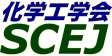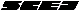## \$B9V1i%W%m%0%i%`!J2q>l!&F|DxJL!K(B

### M\$B2q>l(B \$BBh(B2\$BF|(B

\$B:G=*99?7F|;~!'(B2012-08-20 15:02:17
\$B9V1i(B
\$B;~9o(B
\$B9V1i(B
\$BHV9f(B
\$B9V1iBjL\!?H/I=\$B%-!<%o!<%I(B\$BJ,N`(B
\$BHV9f(B
\$B\$BHV9f(B
\$B%7%s%]%8%&%`(B \$B!c%(%M%k%.!<%7%s%]%8%&%`!d(B
(9:00\$B!A(B10:00)\$B!!(B(\$B:BD9(B \$B?@86(B \$B?.;V(B)
9:00\$B!A(B 9:20M201\$BFqJ,2r@-M-5!J*\$NG.2=3XE*%,%92=FC@-\$N8!F\$(B
(\$BAO2ABg9)(B) \$B!{(B(\$B3X(B)\$BCfEg(B \$B9d;K(B \$B!&(B \$B>>K\(B \$B@6?M(B \$B!&(B (\$B@5(B)\$BEOIt(B \$BAO;N(B \$B!&(B (\$B@5(B)\$B0fED(B \$B=\0l(B \$B!&(B (\$B@5(B)\$B>>;3(B \$BC#(B \$B!&(B (\$B@5(B)\$B;3K\(B \$B1QIW(B
Methane fermentation residue
Waterweed
Gasification
S-2716
9:20\$B!A(B 9:40M202H2\$B\$*\$h\$S(BCO\$B@8@.\$K5Z\$\\$9(BO2/CO2\$B%,%92=\$NFC0[@-(B
(\$BEl9)Bg(B) \$B!{(B(\$B@5(B)\$BEOIt(B \$B90C#(B \$B!&(B Kiatpanachart Dejudom \$B!&(B \$B9S0f(B \$B0jLi(B \$B!&(B (\$B@5(B)\$B2,:j(B \$B7r(B
O2/CO2 coal gasification
detailed chemical reaction
S-2957
9:40\$B!A(B 10:00M203Characteristics of volatile reactions and char gasification in O2/CO2 coal combustion
(Tokyo Inst. Tech.) \$B!{(BLi Xiaofan \$B!&(B Arai Fumiya \$B!&(B Kiatpanachart Dejudom \$B!&(B (\$B@5(B)Watanabe Hirotatsu \$B!&(B (\$B@5(B)Okazaki Ken
O2/CO2 combustion
coal
CO2 reactivity
S-2968
(10:00\$B!A(B11:00)\$B!!(B(\$B:BD9(B \$BLnED(B \$BNh<#(B)
10:00\$B!A(B 10:20M204\$B3lC:MxMQ2PNOH/EE%W%i%s%H\$NG.8zN(8~>eK!\$N8!F\$(B
(\$B4tIlBg1!(B) \$B!{(B(\$B@5(B)\$B?@86(B \$B?.;V(B \$B!&(B (\$B3X(B)\$BIp;3(B \$B>49((B \$B!&(B (\$B@5(B)\$B7(It(B \$BOB90(B \$B!&(B (\$B@5(B)\$B
Low rank coal
Power plant
Thermal efficiency
S-2113
10:20\$B!A(B 10:40M205\$BDcIJ0LC:AG;q8;\$N2~
(\$B5~Bg9)(B) \$B!{(B(\$B3X(B)\$BF#J/(B \$BBgM5(B \$B!&(B (\$B@5(B)\$BiCED(B \$BN40l(B \$B!&(B (\$B@5(B)\$B;01:(B \$B9'0l(B
self-ignition tendency
S-2802
10:40\$B!A(B 11:00M206\$BLZ
(\$B2,;3Bg1!4D6-@8L?(B) \$B!{(B(\$B3X(B)\$BEOJU(B \$BBgCR(B \$B!&(B (\$B@5(B)Uddin Md. Azhar \$B!&(B (\$B@5(B)\$B2CF#(B \$B2E1Q(B
Woody Biomass
Biomass Tar
Catalytic Decomposition
S-2179
(11:00\$B!A(B12:00)\$B!!(B(\$B:BD9(B \$BEOIt(B \$B90C#(B)
11:00\$B!A(B 11:20M207\$B%]%s%]%s>uE44p?(G^\$rMQ\$\$\$?%P%\$%*%^%9M3Mh%?!<%k\$N2~
(\$B90A0BgM}9)(B) \$B!{(B(\$B3X(B)Kaewpanha Malinee \$B!&(B (\$B90A0Bg?7%(%M!<8&(B) (\$B@5(B)\$B41(B \$B9q@6(B \$B!&(B \$B8b(B \$B2BIK(B \$B!&(B (\$B@D?98)9)Am8&(B) (\$B6&(B)\$B3k@>(B \$BM5(B \$B!&(B (\$B90A0Bg?7%(%M!<8&(B) (\$B@5(B)\$B0\$I[(B \$BN\$Ds(B
Biomass tar
Steam reforming
Pompom-like catalyst
S-2112
11:20\$B!A(B 11:40M208\$B%P%\$%*%^%9%?!<%kDc292~
(\$B72Bg(B) \$B!{(B(\$B@5(B)Sirimirin Meesuk \$B!&(B \$B:4F#(B \$B@1EM(B \$B!&(B \$B>>Eh(B \$B:L(B \$B!&(B (\$B@5(B)\$B:4F#(B \$BOB9%(B \$B!&(B (\$B@5(B)\$BJuED(B \$B63G7(B
\$B8GBN?(G^(B
\$B%P%\$%*%^%9(B
\$BDc29%,%92=(B
S-2326
11:40\$B!A(B 12:00M2093\$B<
(\$B72Bg1!9)(B) \$B!{(B(\$B3X(B)\$BD.ED(B \$BM&Lo(B \$B!&(B (\$B72Bg9)(B) \$B=U;3(B \$B9IJ?(B \$B!&(B (\$B72Bg1!9)(B) (\$B@5(B)\$BLnED(B \$BNh<#(B
biomass
bio-fuel
fluidized bed
S-2363
(13:00\$B!A(B14:00)\$B!!(B(\$B:BD9(B \$B5A2H(B \$BN<(B)
13:00\$B!A(B 13:20M213(\$B9V1iCf;_(B)

10036
13:20\$B!A(B 13:40M214\$B3lC:%A%c!x5\$%,%92=\$NB.EYO@\$KBP\$9\$kG.J,2r@-41G=4p\$N1F6A(B
(\$B6eBgC:AG%;(B) \$B!{(B(\$B@5(B)\$B9)F#(B \$B??Fs(B \$B!&(B (\$B6eBgAmM}9)(B) \$BDEF`LZ(B \$B>J8c(B \$B!&(B (\$B6eBg@hF38&(B) (\$B@5(B)\$BB'1J(B \$B9TMG(B \$B!&(B (\$B@5(B)\$BNS(B \$B=a0lO:(B
\$B@PC:(B
\$B%,%92=(B
\$BG.J,2r(B
S-2516
13:40\$B!A(B 14:00M215\$B3lC:!?%P%\$%*%^%9%A%c!<\$NL5?(G^?e>x5\$%,%92=5!9=(B
(\$B6eBg@hF38&(B) \$BH,;3(B \$BLwBe(B \$B!&(B (\$B6eBgC:AG%;(B) \$B!{(B(\$B@5(B)\$B9)F#(B \$B??Fs(B \$B!&(B (\$B6eBg@hF38&(B) (\$B@5(B)\$BB'1J(B \$B9TMG(B \$B!&(B (\$B@5(B)\$BNS(B \$B=a0lO:(B
\$B@PC:(B
\$B%,%92=(B
\$BB.EY<0(B
S-2518
(14:00\$B!A(B15:00)\$B!!(B(\$B:BD9(B \$BB'1J(B \$B9TMG(B)
14:00\$B!A(B 14:20M216\$BD>@\DLEE>.7?H?1~AuCV\$rMQ\$\$\$?9b299b05>r7o\$G\$N@PC:%,%92=H?1~B.EYB,Dj(B
(\$B5~Bg9)(B) \$B!{(B(\$B3X(B)\$B:#0f(B \$B=SJe(B \$B!&(B (\$B@5(B)\$B:{2,(B \$B1Q;J(B \$B!&(B (\$B@5(B)\$BiCED(B \$BN40l(B \$B!&(B (\$B@5(B)\$B;01:(B \$B9'0l(B
coal gasification
directly-heated reactor
Langmuir-Hinshelwood type equation
S-2796
14:20\$B!A(B 14:40M217Air gasification of biomass pellets in the auto-thermal packed bed reactor
(\$BL>Bg1!9)(B) \$B!{(B(\$B3X(B)Khedu Joseph \$B!&(B (\$BL>Bg%(%3(B) \$BI[L\(B \$BM[;R(B \$B!&(B (\$B@5(B)\$B?"LZ(B \$BJ]><(B \$B!&(B (\$BL>Bg1!9)(B) (\$B@5(B)\$B5A2H(B \$BN<(B \$B!&(B (\$BL>Bg%(%3(B) (\$B@5(B)\$B@.@%(B \$B0lO:(B
biomass
gasification
auto-thermal
S-2155
14:40\$B!A(B 15:00M218High Temperature Gasification of Plastic Waste
(\$BL>Bg1!9)(B) \$B!{(B(\$B3X(B)Nedjalkov Ivan Jordanov \$B!&(B (\$B@5(B)\$B5A2H(B \$BN<(B \$B!&(B (\$BL>Bg%(%3%H%T%"(B) (\$B@5(B)\$B?"LZ(B \$BJ]><(B \$B!&(B (\$B@5(B)\$B@.@%(B \$B0lO:(B \$B!&(B (\$B@5(B)\$BI[L\(B \$BM[;R(B
gasification
plastic
tar
S-2533

\$B9V1i%W%m%0%i%`(B
\$B2=3X9)3X2q(B \$BBh(B44\$B2s=)5(Bg2q(B(C) 2012 \$B8x1W
Most recent update: 2012-08-20 15:02:17
For more information contact \$B2=3X9)3X2qElKL;YIt(B \$BBh(B44\$B2s=)5(Bg2q(B \$BLd\$\$9g\$;78(B
E-mail: inquiry-44fwww3.scej.org
This page was generated byeasp 2.28; proghtml 2.28c (C)1999-2011 kawase